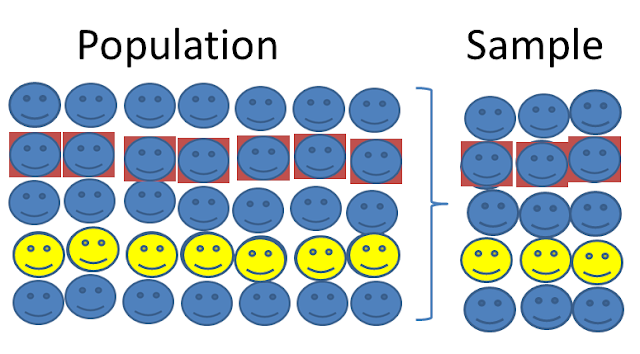# Population vs Sample in Statistics

Population vs sample in statistics are plays two different role in statistics. Population is the whole study area where sample is the required study area from the whole study area.Population vs Sample-statistical aid

### Population

Population indicates the all data or whole observations under a particular study area or a study variable. That means , a set of similar items or events or data is defined by population.
For example-
If we choose a particular area or subject for our study or research purpose , then we have to collect all the information related to that subject or area. Here all the collected information is our population.
That means ,
Suppose , we want to study about educational qualification of Bangladeshi people. so here all the Bangladeshi people is our population under our study variable educational qualification.

### Sample

A representative part of population is defined by sample. That means , a sample is a subset of population which contains all the feature or attributes of population.For above example we can say ,
sample=peoples of Khulna divisionBecause , Khulna division included in Bangladesh                                                                      & people of Khulna division is also exist in Bangladesh.

Sometimes ,
population=sample

*parameter=population characteristics
*statistic=sample characteristics

Statistic: characteristics or function of sample is defined by statistic and where the function is independent. sometimes , it is defined by the value of that function .
For example,
let we have 5 sample(20,21,23,25,23) which indicates the age (in year) of 5 students of  a college.
then we want to calculate the arithmetic mean for this sample,so, A.M=(20+21+23+25+23)/5
= 22.5
here A.M or sample mean is called statistic.